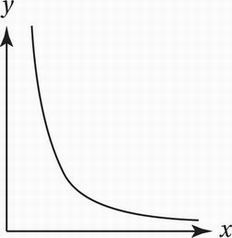# SAT Math Multiple Choice Question 709: Answer and Explanation

### Test Information

Question: 709

4. Which of the following scatterplots provides the strongest evidence in support of the hypothesis that y varies inversely as the square of x?

• A.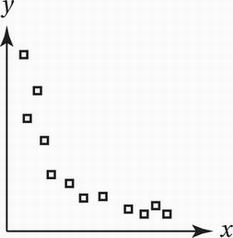• B.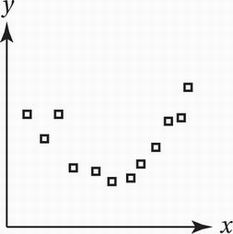• C.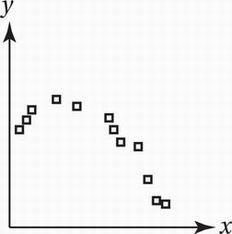• D.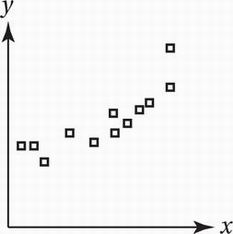If y varies inversely as the square of x, then the variables are related by the equation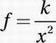, where k is a positive constant. The graph of such an equation in the xy-plane looks like this: